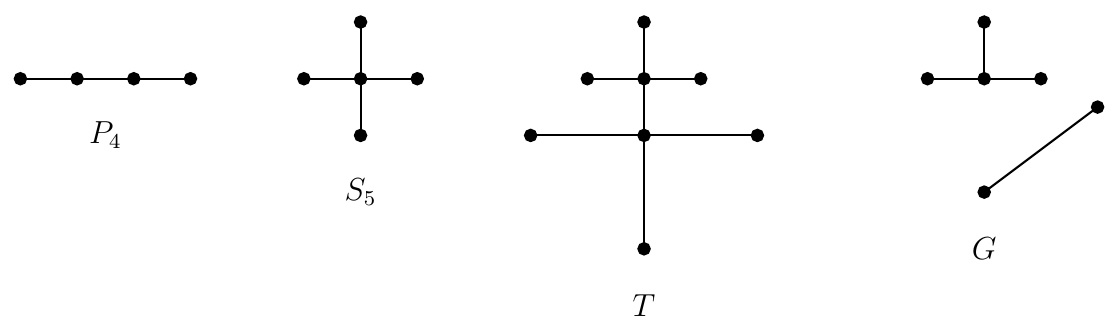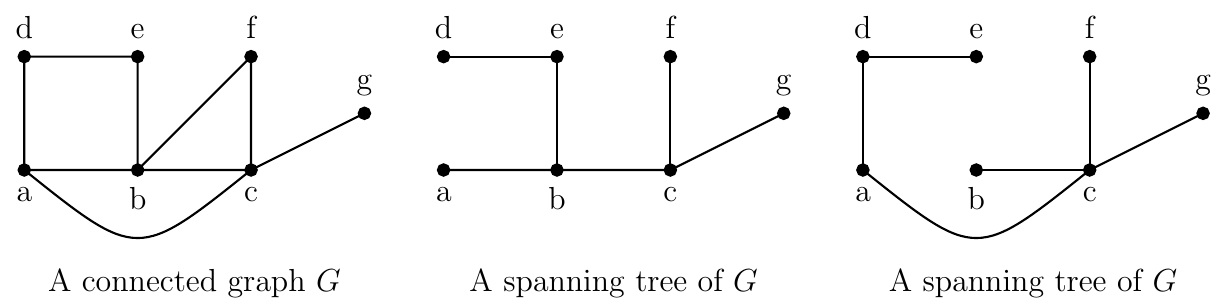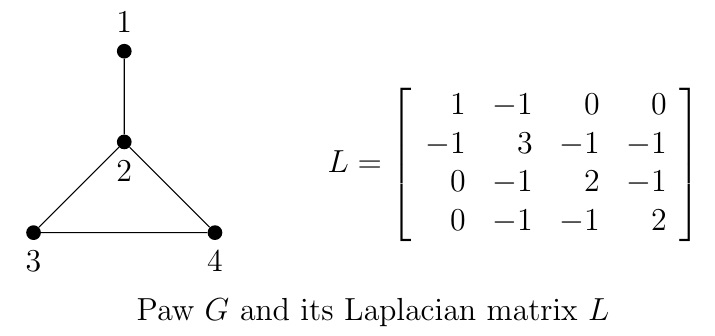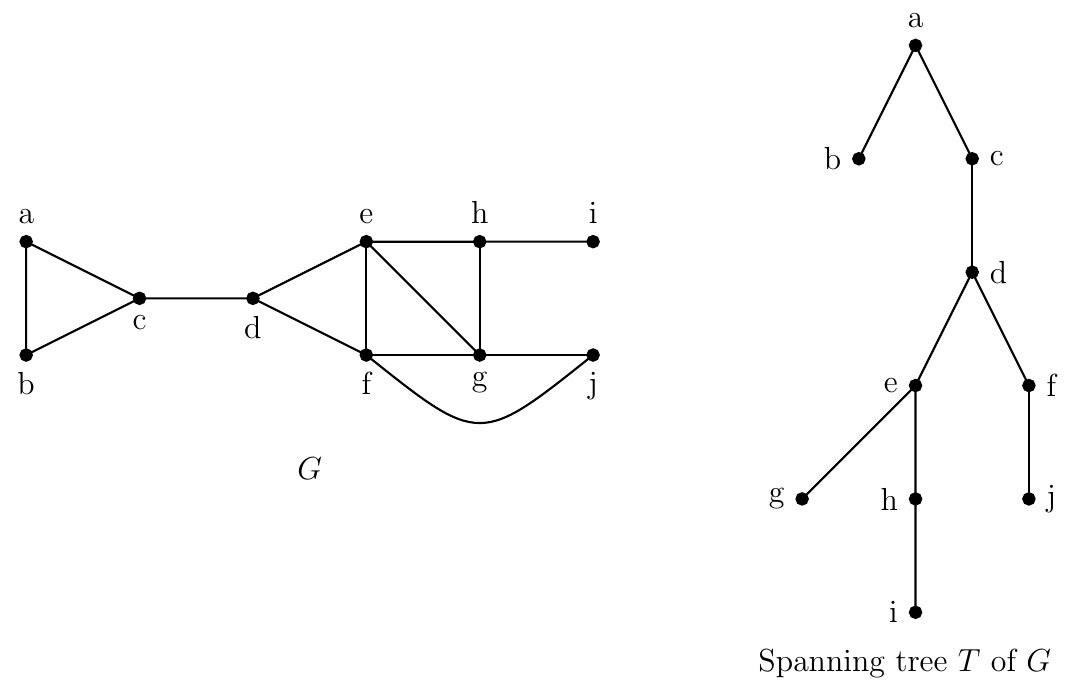Discrete Math Home

## Trees

Definition (Tree). A tree is a simple connected graph with no cycles. A graph is a forest if each of its connected components is a tree.Example. $$P_4,\;S_5$$, and $$T$$ are trees and $$G$$ is a forest.

Remark. In 1857, Aurther Caeley used trees to enumerate the isomers of saturated hydrocarbons $$C_nH_{2n+2}$$. For example, butane $$C_4H_{10}$$ has two isomers and they look like trees where atoms and atomic bonds represent vertices and edges respectively. Applications of trees include organizing a sequence of decisions and creating file directories in a computer and least expensive communication network.

Theorem. Let $$G$$ be a simple graph on $$n$$ vertices. Then the following are true.

1. $$G$$ is a tree if and only if $$G$$ is a connected graph with $$n-1$$ edges.

2. $$G$$ is a tree if and only if there is a unique path between any two vertices in $$G$$.

(a) By induction on $$n$$.

(b) Suppose there is a unique path between any two vertices in $$G$$. Then $$G$$ is connected. Conversely suppose that $$G$$ is a tree. Then $$G$$ is connected and consequently there is a path between any two vertices in $$G$$. If there is more than one path between two vertices in $$G$$, then $$G$$ would have a cycle. Since $$G$$, being a tree, has no cycles, there is a unique path between any two vertices in $$G$$.

Corollary. A tree $$T$$ with at least 2 vertices is a bipartite graph and hence $$\chi(T)=2$$.

Fix a vertex $$v$$ in $$T$$ and color it red. Color vertex $$u$$ blue if the unique path from $$v$$ to $$u$$ has odd length and red otherwise. Since there is no cycle in $$T$$, there is no edge between two blue vertices or two red vertices. Thus blue vertices and red vertices form two partite sets of the bipartite graph $$T$$ and consequently $$\chi(T)=2$$.

Definition (Spanning tree). A spanning tree of a graph $$G$$ is a subgraph that is a tree containing all the vertices of $$G$$. A spanning forest of a graph $$G$$ is a subgraph that is a forest containing all the vertices of $$G$$.There may be a lot of spanning trees of a connected graph. Kirchhoff's theorem or matrix tree theorem provides the number of spanning trees in terms of nonzero eigenvalues of the Laplacian matrix of a graph.

Theorem (Matrix tree theorem). Let $$G$$ be a simple graph on $$n$$ vertices and $$L$$ be the Laplacian matrix of $$G$$ with eigenvalues $$0=\mu_1\leq \mu_2\leq \cdots \leq \mu_n$$. Then the number $$t(G)$$ of spanning trees of $$G$$ is $t(G)=\det\left(L(i)\right)=\frac{\mu_2\cdot \mu_3 \cdots \mu_n}{n},$ for all $$i=1,2,\ldots,n$$.Example. The Laplacian matrix $$L$$ of the paw graph $$G$$ has eigenvalues $$0,1,3,4$$. By matrix tree theorem, the number of its spanning trees is $\det\left(L(2)\right)=\det \left[\begin{array}{rrr} 1&0&0\\ 0&2&-1\\ 0&-1&2 \end{array}\right]=\frac{1\cdot 3 \cdot 4}{4}=3.$ Remark. How do we find a spanning tree of a connected graph? Choose a cycle and delete one edge. Do it for all cycles, one at a time.

Problem. Find the number of edges whose removal from a connected graph $$G$$ on $$n$$ vertices and $$m$$ edges produces a spanning tree of $$G$$.

Solution. Let $$x$$ be the required number of edges to be deleted to get a spanning tree of a connected graph $$G$$ on $$n$$ vertices and $$m$$ edges. Since a spanning tree on $$n$$ vertices has $$n-1$$ edges, we have $$m-x=n-1$$. Thus $$x=m-n+1$$.

Theorem. A graph $$G$$ is connected if and only if $$G$$ has a spanning tree.

($$\Longleftarrow$$) Suppose that $$G$$ has a spanning tree $$T$$. Then there a path between any two vertices of $$T$$, hence of $$G$$.

($$\Longrightarrow$$) Suppose $$G$$ is connected. If $$G$$ has no cycles, $$G$$ is a tree and hence a spanning tree of itself. Otherwise $$G$$ has a cycle and delete one of its edges, say $$e$$. Then $$G-e$$ is still connected. By deleting edges of cycles of $$G$$, one at a time, we end up with a connected graph with no cycles which is a spanning tree of $$G$$.

Deleting edges from cycles to produce a spanning tree is inefficient as we have to identify cycles at each step. There are two better algorithms: depth-first search (backtracking) and breadth-first search. We illustrate breadth-first search by an example. First we need the following definition.

Definition (Rooted tree). A rooted tree is a tree in which one vertex is specified as the root. The level of a vertex $$v$$ in a rooted tree is the length of the unique path from $$v$$ to the root.Problem. Use breadth-first search to produce a spanning tree $$T$$ for the above graph $$G$$. Choose $$a$$ as the root of $$T$$.

Solution. Start with vertex $$a$$, the root. For the level 1 vertices, choose all the neighbors of $$a$$ and add edges joining $$a$$ and its neighbors. So we get $$b$$ and $$c$$ as the level 1 vertices (in alphabetical order) and edges $$\{a,b\}$$ and $$\{a,c\}$$.

For the level 2 vertices, choose all the neighbors of $$b$$ and $$c$$, that are not already chosen, and order them (alphabetically for this problem). For this graph, $$d$$ is the only vertex in level 2. Join the first vertex in level 1, i.e., $$b$$, and its neighbors in level 2. $$b$$ is not adjacent to the only vertex $$d$$ in level 2. Then join the second vertex in level 1, i.e., $$c$$, and its neighbors in level 2 that are not already chosen. So we get $$\{c,d\}$$.

For the level 3 vertices, choose all the neighbors of $$d$$, that are not already chosen, and add edges joining $$d$$ and its neighbors in level 3. So we get $$e$$ and $$f$$ as the level 3 vertices (in alphabetical order) and edges $$\{d,e\}$$ and $$\{d,f\}$$.

For the level 4 vertices, choose all the neighbors of $$e$$ and $$f$$, that are not already chosen, and order them. We get $$g$$, $$h$$, and $$j$$ as the level 4 vertices. Join the first vertex in level 3, i.e., $$e$$, and its neighbors in level 4. So we get $$\{e,g\}$$ and $$\{e,h\}$$. Then join the second vertex in level 3, i.e., $$f$$, and its neighbors in level 4 that are not already chosen. So we get $$\{f,j\}$$.

Continuing this process, we get $$\{h,i\}$$ and a tree $$T$$. Since tree $$T$$ contains all the vertices of $$G$$, $$T$$ is a spanning tree of $$G$$.

Last edited